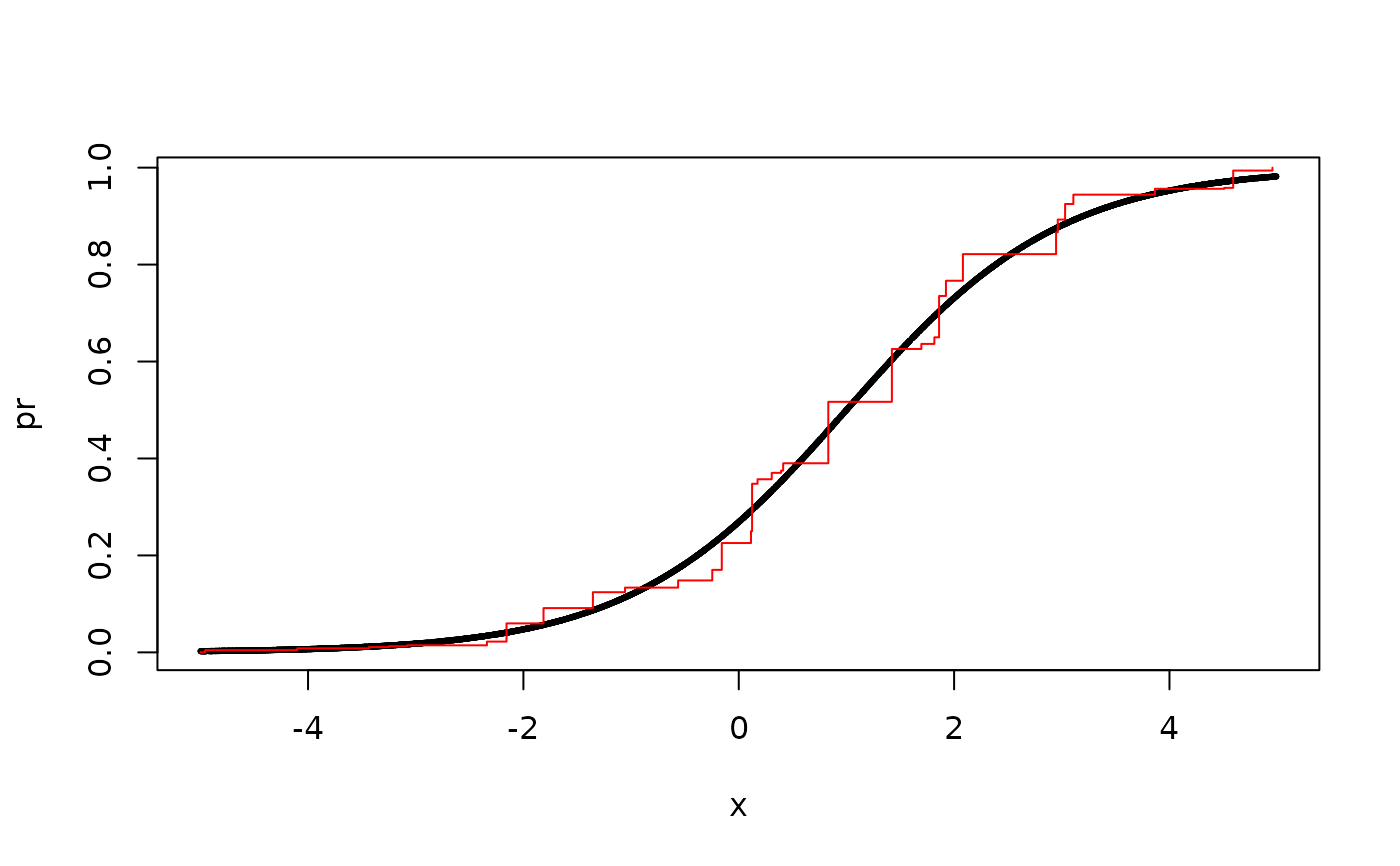pava(y, x = numeric(0), weights = numeric(0))

## Arguments

y

response variable

x

(optional) predictor vector (otherwise y is assumed to be a priori sorted according to relevant predictor)

weights

weights (optional) weights

## Value

List with index (idx) of jump points and values (value) at each jump point.

Klaus K. Holst

## Examples

x <- runif(5e3, -5, 5)
pr <- lava::expit(-1 + x)
y <- rbinom(length(pr), 1, pr)
pv <- pava(y, x)
plot(pr ~ x, cex=0.3)
with(pv, lines(sort(x)[index], value, col="red", type="s"))